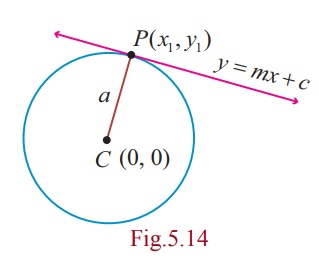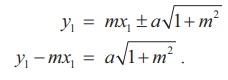Home | | Maths 12th Std | Condition for the line y = mx + c to be a tangent to the circle and finding the point of contact

# Condition for the line y = mx + c to be a tangent to the circle and finding the point of contact

Condition for the line y = mx + c to be a tangent to the circle and finding the point of contact

Condition for the line y = mx + c to be a tangent to the circle x2 + y2 = a2 and finding the point of contact

Let the line y = mx + c touch the circle x2 + y2 = a2 . The centre and radius of the circle x2 + y2 = a2 are (0, 0) and a respectively.

## (i) Condition for a line to be tangent

Then the perpendicular distance of the line y mx c = 0 from (0, 0) isThis must be equal to radius .Therefore= a or c2 = a2 (1+ m2) .

Thus the condition for the line y = mx + c to be a tangent to the circle x2 + y2 = a2 is c2 = a2 (1 + m2).

## (ii) Point of contact

Let (x1 , y1 ) be the the point of contact of y = mx + c with the circle x2 + y2 = a2,Then  y1 =  mx1 + c       ……….(1)

Equation of tangent at (x1 , y1 ) is xx1 + yy1 = a2 .

yy1  =  -xx1 + a2       ... (2)

Equations (1) and (2) represent the same line and hence the coefficients are proportional.Then the points of contact is eitherNote

The equation of tangent at P to a circle is y = mx ± a √[1 +m2]

Theorem 5.4

From any point outside the circle x2 + y2 = a2 two tangents can be drawn.

Proof

Let P(x1 , y1 ) be a point outside the circle. The equation of the tangent isIt passes through (x1, y1) . ThereforeSquaring both sides, we get

( y1 – mx1)2 = a2 (1+ m2 )

y12 + m2 x12 - 2mx1y1 - a2 - a2m2 = 0

m2 (x12 - a2 ) - 2mx1y1 + ( y12 - a2 ) = 0 .

This quadratic equation in m gives two values for m .

These values give two tangents to the circle x2 + y2 = a2.

Note

(1)  If (x1, y1 ) is a point outside the circle, then both the tangents are real.

(2)  If (x1, y1 ) is a point inside the circle, then both the tangents are imaginary.

(3)  If (x1, y1 ) is a point on the circle, then both the tangents coincide.

Example 5.11

Find the equations of the tangent and normal to the circle x2 + y2 = 25 at P(−3, 4) .

Solution

Equation of tangent to the circle at P(x1 , y1 ) is xx1 + yy1 = a2

That is, x(-3) + y(4) = 25

-3x + 4 y = 25

Equation of normal is xy1 - yx1 = 0

That is, 4x + 3y = 0 .

Example 5.12

If y = 4x + c is a tangent to the circle x2 + y2 = 9 , find c.

Solution

The condition for the line y = mx + c to be a tangent to the circle x2 + y2 = a2 is c2 = a2 (1+ m2).

Then, c = ± √[9(1+16)]

c = ±3 √17.

Example 5.13

A road bridge over an irrigation canal have two semi circular vents each with a span of 20m and the supporting pillars of width 2m . Use Fig.5.16 to write the equations that represent the semi-verticular vents.Solution

Let  O1 O2  be  the  centres  of  the two semi circular vents.

First vent with centre O1 (12, 0) and radius r = 10 yields equation to first semicircle as

(x -12)2 + ( y - 0)2 = 102

Þ x2 + y2 - 24x + 44 = 0 , y > 0 .

Second vent with centre O2 (34, 0) and radius r = 10 yields equation to second vent as

(x - 34)2 + y2 = 102

Þ x2 + y2 - 68x +1056 = 0 , y > 0 .

Tags : Formula, Solved Example Problems , 12th Mathematics : UNIT 5 : Two Dimensional Analytical Geometry II
Study Material, Lecturing Notes, Assignment, Reference, Wiki description explanation, brief detail
12th Mathematics : UNIT 5 : Two Dimensional Analytical Geometry II : Condition for the line y = mx + c to be a tangent to the circle and finding the point of contact | Formula, Solved Example Problems